# Time and Date Calculation in Microsoft Excel 2010

In this article, we will learn how to calculate the Time and Date in Microsoft Excel 2010.

To return the time and date, we can use the Date, Time, Today and Now functions in Microsoft Excel.

To enter a date in Excel use the “/” or “-“character and to enter the time we use “ : “. Also, we can use formulas and shortcut keys.

If we have year, month and day in 3 different cell then we can write the formula and select the cells.

Excel Date formula will be used to convert the number that represents the date as date in Microsoft Excel date-time code.

The Syntax of Date:- =Date (year, month, date)

For Example:- Year in cell A2, month in cell B2 and date in cell C2.

• Write the formula in cell D2 =DATE(A2,B2,C2).
• Press Enter on your keyboard.
• The function will convert it into date.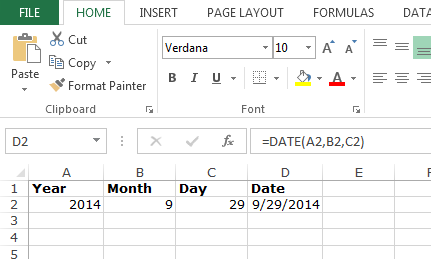Excel Time formula is used to convert hours, minutes, and seconds given as number to an Excel serial number, formatted with a time format.

The Syntax of Time:- =Time(Hour, minute, Seconds)

For Example:- Hour in cell A2, minutes in cell B2 and seconds in cell C2.

• Write the formula in cell D2 =Time(A2,B2,C2).
• Press Enter on your keyboard.
• The function will convert it into Time.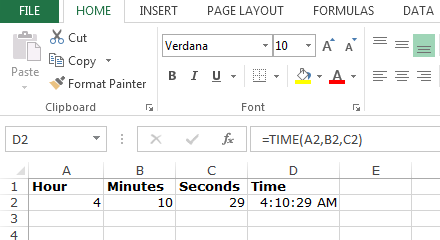To enter the today date, use Today Function.

Today function is used to return the current date formatted as a date.

The Syntax of Today:- =Today()

For Example:-

• Write the formula in cell A1.
• =Today(), press Enter on your keyboard.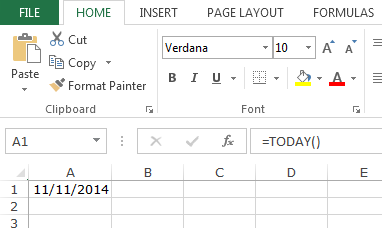We can use shortcut key to return the current date.

• Select the cell A1.
• Press the key Ctrl+; then press Enter on your keyboard.
• Today date will show.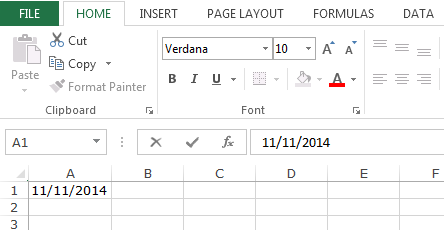We can add current date and time by using short keys in excel.

Excel Now function is used to return the current date and time formatted as a date.

The Syntax of NOW:- =NOW()

For Example:-

• Write the formula in cell A1.
• =NOW(), press Enter on your keyboard.
• The function will return Current date and time.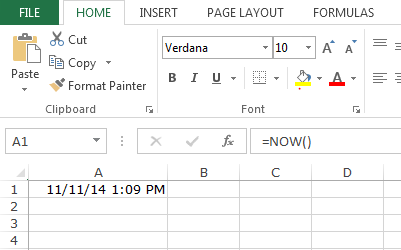We can add current date and time by using shortcut keys in Excel.

• Select the cell A1.
• Select a cell and press CTRL+; then SPACE, and then CTRL+SHIFT+;
• Press Enter on your keyboard.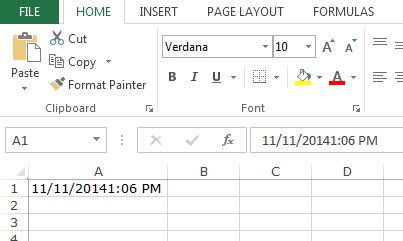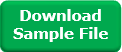1.